## Exercises

1. Characterize the stability at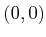of the vector field on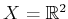, given by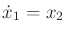and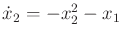. Use the Lyapunov function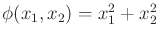.
2. Repeat Example 15.4, but instead use the cost term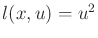.
3. Repeat Example 15.4, but instead for a triple integrator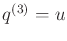and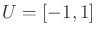.
4. Determine the precise conditions under which each of the four cases of Example 15.4 occurs. Define a feedback motion plan that causes time-optimal motions.
5. Note that some of the six optimal words for the Dubins car do not appear for the Reeds-Shepp car. For each of these, illustrate why it does not appear.
6. Retrace the steps of the Taylor series argument for deriving the Lie bracket in Section 15.4.2. Arrive at (15.81) by showing all steps in detail (smaller steps are skipped in Section 15.4.2).
7. Determine whether the following system is nonholonomic and STLC: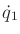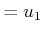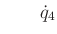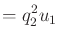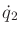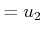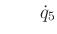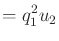(15.166)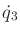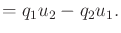8. Prove that linear systems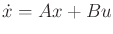for constant matrices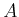and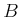cannot be nonholonomic.
9. Determine whether the following system is nonholonomic and STLC: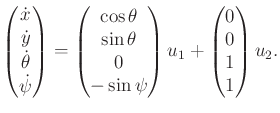(15.167)

10. Using the commutator motion and constant actions for the differential drive, develop a lattice over its configuration space.
11. Consider a smooth nonlinear system that has only one action variable and an-dimensional state space for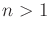. Are such systems always completely integrable, always nonholonomic, or is either possible?
12. Generalize Example 15.17 to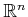with two action variables. Determine whether the system is STLC for any.
13. Show that the vector cross product on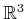indeed produces a Lie algebra when used as a bracket operation.
14. Derive the CFS equation for the following system: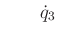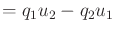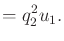(15.168)

Implementations

15. Implement software that computes the P. Hall basis up to any desired order (this is only symbolic computation; the Lie brackets are not expanded).
16. Implement software that displays the appropriate optimal path for the Dubins car, between any given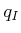and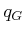.
17. Apply the planning algorithm in Section 14.4.2 to numerically determine the Dubins curves. Use Dijkstra's algorithm for the search, and use a high-resolution grid. Can your software obtain the same set of curves as Dubins?
18. Experiment with using Dubins curves as a local planning method (LPM) and metric in an RRT-based planning algorithm. Does using the curves improve execution time? Do they lead to better solutions?

Steven M LaValle 2020-08-14